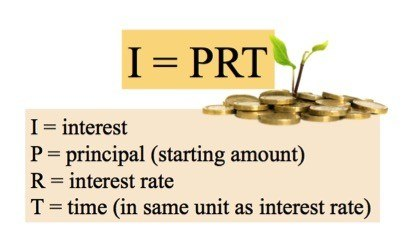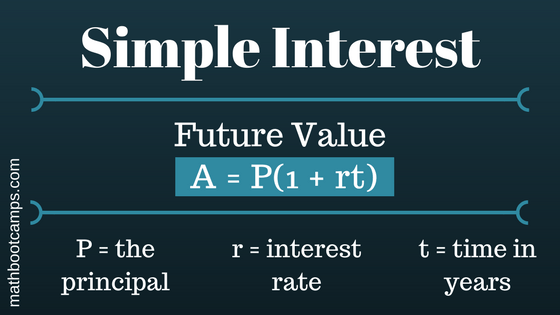# FINANCIAL MATHEMATHICAL LITERACY ( SIMPLE INTEREST)

Introduction

### THE MOST THING TO KNOW IN ORDER TO CALCULATE THE SIMPLE INTEREST IS TO KEEP IN MIND THE FORMULA USED IN THE BELOW PICTURE:So the formula for working out interest is

• I = P x R x T

As an easy example, consider how much interest MR MOYO will get by investing R10 000 at a rate of 10% over a period of one year.

I = P x R x T

I = R10 000 x 10% x 1

I = R1000

SO R1000 IS THE SIMPLE INTEREST THAT WILL BE RECEIVED.

## Finding the future value for simple interest

One way to calculate the future value would be to just find the interest and then add it to the principal. The quicker method however, is to use the following formula.You will also be required to calculate the investment/loan, interest rate and years.

The Simple Interest Pyramid below shows how we can manipulate the variablesWHEN YOU WANT TO CALCULATE THE PRINCIPLE AMOUNT WE USE THE FIRST FORMULA

WHEN YOU WANT TO CALCULATE THE RATE WE USE THE SECOND FORMULA

WHEN YOU WANT TO CALCULATE THE NUMBER OF TIME YOU USE THE THIRD FORMULA

Interest Rate

• R = I / (P)(T)

Investment

• P = I / (R)(T)

Years

• T = I / (P)(R)

A total of \$1,200 is invested at a simple interest rate of 6% for 4 months. How much interest is earned on this investment?

A 2-year loan of \$500 is made with 4% simple interest. Find the interest earned.

A business takes out a simple interest loan of \$10,000 at a rate of 7.5%. What is the total amount the business will repay if the loan is for 8 years?

Process

EXAMPLE 1

A 2-year loan of \$500 is made with 4% simple interest. Find the interest earned.

EXAMPLE 2

A total of \$1,200 is invested at a simple interest rate of 6% for 4 months. How much interest is earned on this investment?

Evaluation

Before we can apply the formula, we will need to write the time of 4 months in terms of years. Since there are 12 months in a year:

t=412=13

With this adjusted to years, we can now apply the formula with P=1200

and r=0.06

.

I=Prt=1200(0.06)(13)=24

Answer: The interest earned is \$24.

Always take a moment to identify the values given in the problem. Here we are given:

• Time is 2 years: t=2
•
• Initial amount is \$500: P=500
•
• The rate is 4%. Write this as a decimal: r=0.04
•

Now apply the formula:

I=Prt=500(0.04)(2)=40

Answer: The interest earned is \$40.

The total amount they will repay is the future value, A

. We are also given that:

• t=8
•
• r=0.075
•
• P=10000
•

Using the simple interest formula for future value:

A=P(1+rt)=10000(1+0.075(8))=16000

Conclusion

Important to remember that:

Interest is the amount of money added to a investment or loan.

Simple Interest is interest calculated only on the initial amount that you invested or borrowed.

## Continue your study of interest

Now that you have studied the simple interest formula, you can learn the more advanced idea of compound interest. Most savings accounts, credit cards, and loans are based on compound instead of simple interestCredits

Teacher Page